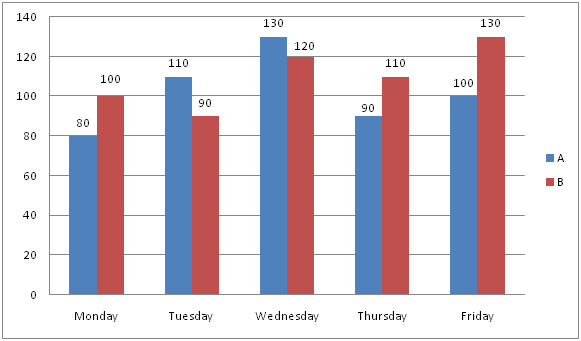# LIC AAO/SBI PO Prelims Quantitative Aptitude Questions 2019 (Day-19)

Dear Aspirants, Our IBPS Guide team is providing new series of Quantitative Aptitude Questions for LIC AAO/SBI PO 2019 so the aspirants can practice it on a daily basis. These questions are framed by our skilled experts after understanding your needs thoroughly. Aspirants can practice these new series questions daily to familiarize with the exact exam pattern and make your preparation effective.

[WpProQuiz 5831]

### Click Here for SBI PO Pre 2019 High-Quality Mocks Exactly on SBI Standard

Directions (Q. 1 – 5): what value should come in place of (?) in the following questions?

1) 48 % of 1600 – 123 + (3/8) of 5680 =? + 112

a) 1058

b) 1372

c) 1526

d) 1844

e) None of these

2) 12 × 564 + 452 – 12 % of 150 = ?2 + 126

a) 105

b) 112

c) 93

d) 98

e) None of these

3) 3 (¾) + 4 (1/8) – 5 (½) + 2 (¼) = ? – 1 (1/8)

a) 4 (7/8)

b) 6 (½)

c) 7 (¼)

d) 5 (¾)

e) None of these

4) ? % of 800 = 1262 – (322 – 162) × 27 ÷ 72

a) 135.25

b) 121.75

c) 116.5

d) 104.5

e) None of these

5) (11 × 11)4 ÷ (1331 ÷ 11)2 × (121 × 11)3 = (11)? + 5

a) 9

b) 11

c) 8

d) 13

e) None of these

Directions (Q. 6 – 10): Study the following information carefully and answer the given questions.

The following line graph shows the total kilocalories burned by 2 individuals (P and Q) on treadmill in 5 different days in a certain week.6) If the total kilocalories burned by P and Q are increased by 10 % and 20 % respectively from Friday to Saturday, then find the ratio between the total kilocalories burned by P in Wednesday and Saturday together to that of total kilocalories burned by Q in Thursday and Saturday together?

a) 20: 23

b) 15: 17

c) 6: 11

d) 35: 43

e) None of these

7) Find the difference between the total kilocalories burned by P to that of Q in all the given days together?

a) 60

b) 90

c) 50

d) 40

e) None of these

8) Total number of kilocalories burned by P in Monday, Tuesday and Friday together is approximately what percentage more/less than the total kilocalories burned by Q in Wednesday, Thursday and Friday together?

a) 20 % more

b) 20 % less

c) 15 % less

d) 30 % more

e) 30 % less

9) If the average kilocalories burned by P in Monday, Wednesday, Friday and Saturday together is 115, then find the total kilocalories burned by P in Saturday?

a) 150

b) 140

c) 125

d) 135

e) None of these

10) Total kilocalories burned by P in Monday and Tuesday together is approximately what percentage of total kilocalories burned by Q in Wednesday?

a) 145 %

b) 125 %

c) 160 %

d) 110 %

e) 175 %

Direction (1-5) :

48 % of 1600 – 123 + (3/8) of 5680 = x + 112

(48/100)*1600 – 1728 + (3/8)*5680 – 112 = x

X = 768 – 1728 + 2130 – 112 = 1058

12 × 564 + 452 – 12 % of 150 = x2 + 126

6768 + 2025 – (12/100)*150 = x2 + 126

6768 + 2025 – 18 – 126 = x2

= > x2 = 8649

= > x = 93

3 (¾) + 4 (1/8) – 5 (½) + 2 (¼) + 1 (1/8) = x

X = (3 + 4 – 5 + 2 + 1) (3/4 + 1/8 – ½ + ¼ + 1/8)

X = 5 [(6 + 1 – 4 + 2 + 1)/8] = 5 (¾)

(x/100)*800 = 1262 – [(32 + 16) (32 – 16) × 27 ÷ 72]

8x = 1262 – [(48*16*27)/72]

8x = 1262 – 288

8x = 974

X = (974/8) = 121.75

118 ÷ (112)2 × (112 × 11)3 = (11)x + 5

118 ÷ 114 × 119 = (11)x + 5

118 – 4 + 9 = 7x + 5

1113 = 7x + 5

13 = x + 5

= > x = 8

Direction (6-10) :

The total kilocalories burned by P in Saturday

= > 100*(110/100) = 110

The total kilocalories burned by Q in Saturday

= > 130*(120/100) = 156

The total kilocalories burned by P in Wednesday and Saturday together

= > 130 + 110 = 240

The total kilocalories burned by Q in Thursday and Saturday together

= > 120 + 156 = 276

Required ratio = 240: 276 = 20: 23

The total kilocalories burned by P in all the given days together

= > 80 + 110 + 130 + 90 + 100 = 510

The total kilocalories burned by Q in all the given days together

= > 100 + 90 + 120 + 110 + 130 = 550

Required difference = 550 – 510 = 40

Total kilocalories burned by P in Monday, Tuesday and Friday together

= > 80 + 110 + 100 = 290

The total kilocalories burned by Q in Wednesday, Thursday and Friday together

= > 120 + 110 + 130 = 360

Required % = [(360 – 290)/360]*100 = 19.44 % = 20 % less

The average kilocalories burned by P in Monday, Wednesday, Friday and Saturday together = 115

The total kilocalories burned by P in Monday, Wednesday, Friday and Saturday together = 115*4 = 460

The total kilocalories burned by P in Saturday

= > 460 – (80 + 130 + 100)

= > 460 – 310 = 150

Total kilocalories burned by P in Monday and Tuesday together

= > 80 + 110 = 190

Total kilocalories burned by Q in Wednesday = 120

Required % = (190/120)*100 = 158.33 % = 160 %